# RD Sharma Solutions for Class 9 Maths Chapter 12 Heron's Formula Exercise 12.1

RD Sharma Solutions for Class 9 Maths are given here for Chapter 12 exercise 12.1. In this exercise, students will learn how to find the area of a triangle using heron’s formula with the help of various solved examples.

Heron’s Formula: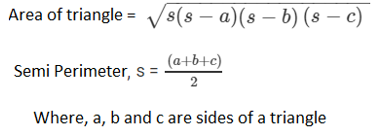The Solutions for RD Sharma class 9 Maths chapter 12 exercise 12.1 includes solved problems and step by step explanations given by our experts to further helps students find alternative ways to solve a problem.

## Download PDF of RD Sharma Solutions for Class 9 Maths Chapter 12 Heron’s Formula Exercise 12.1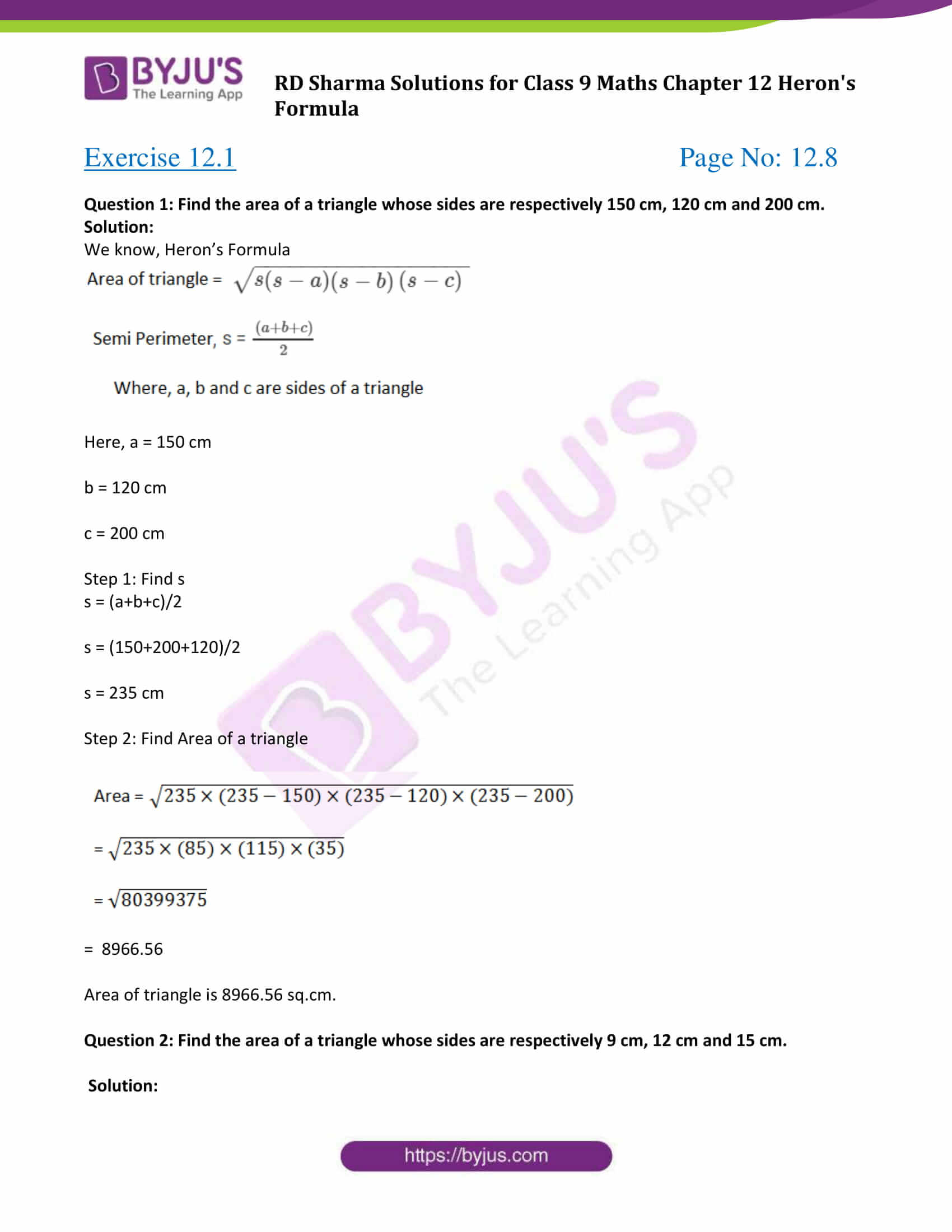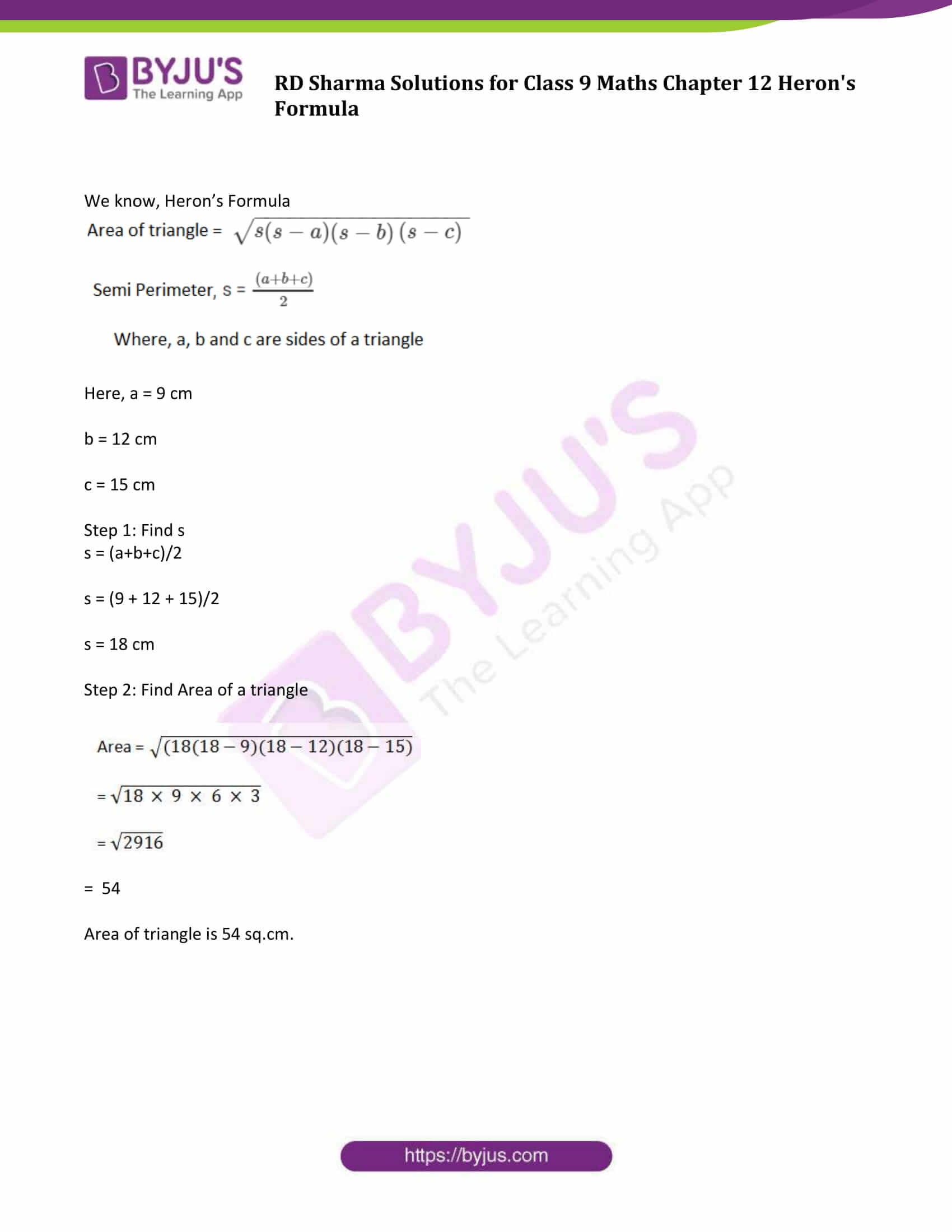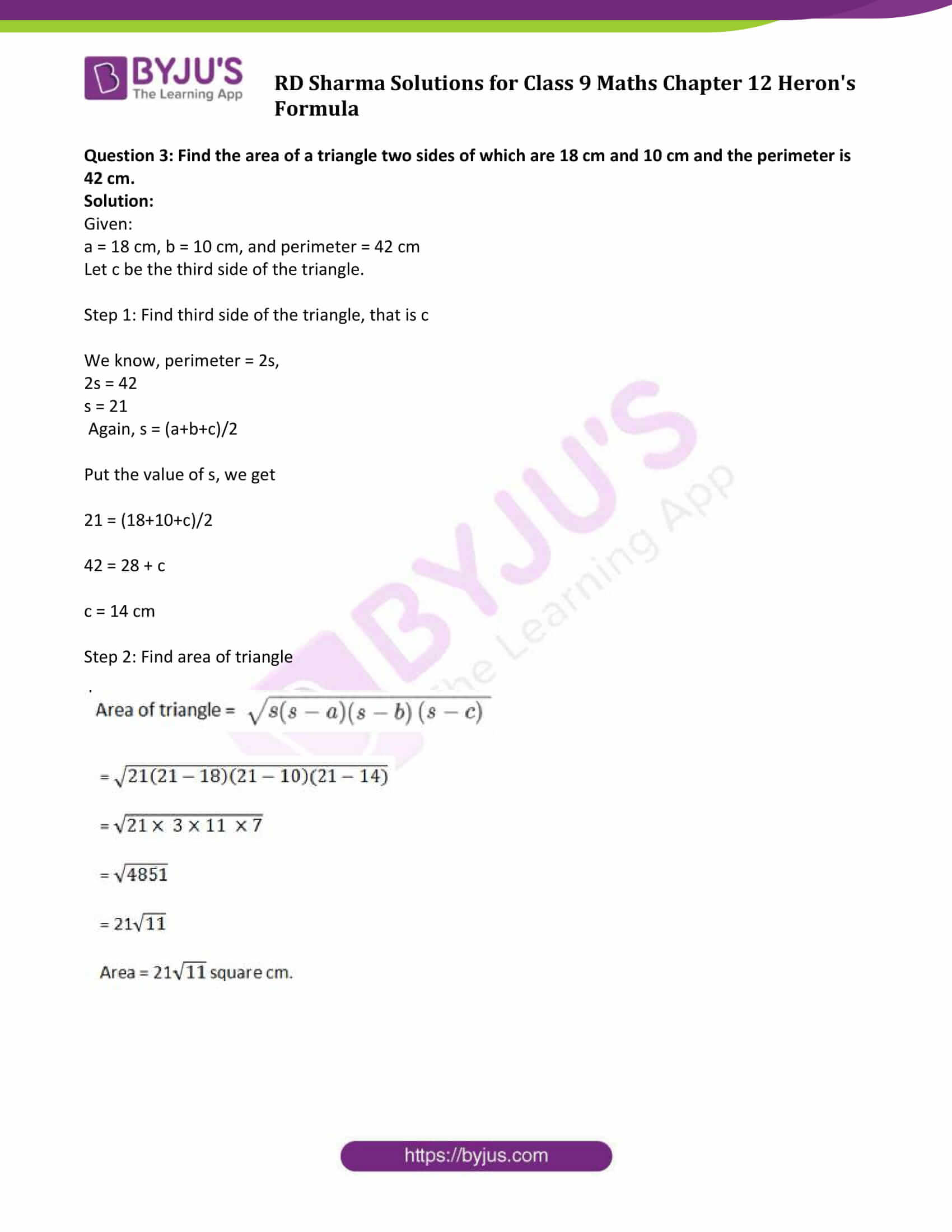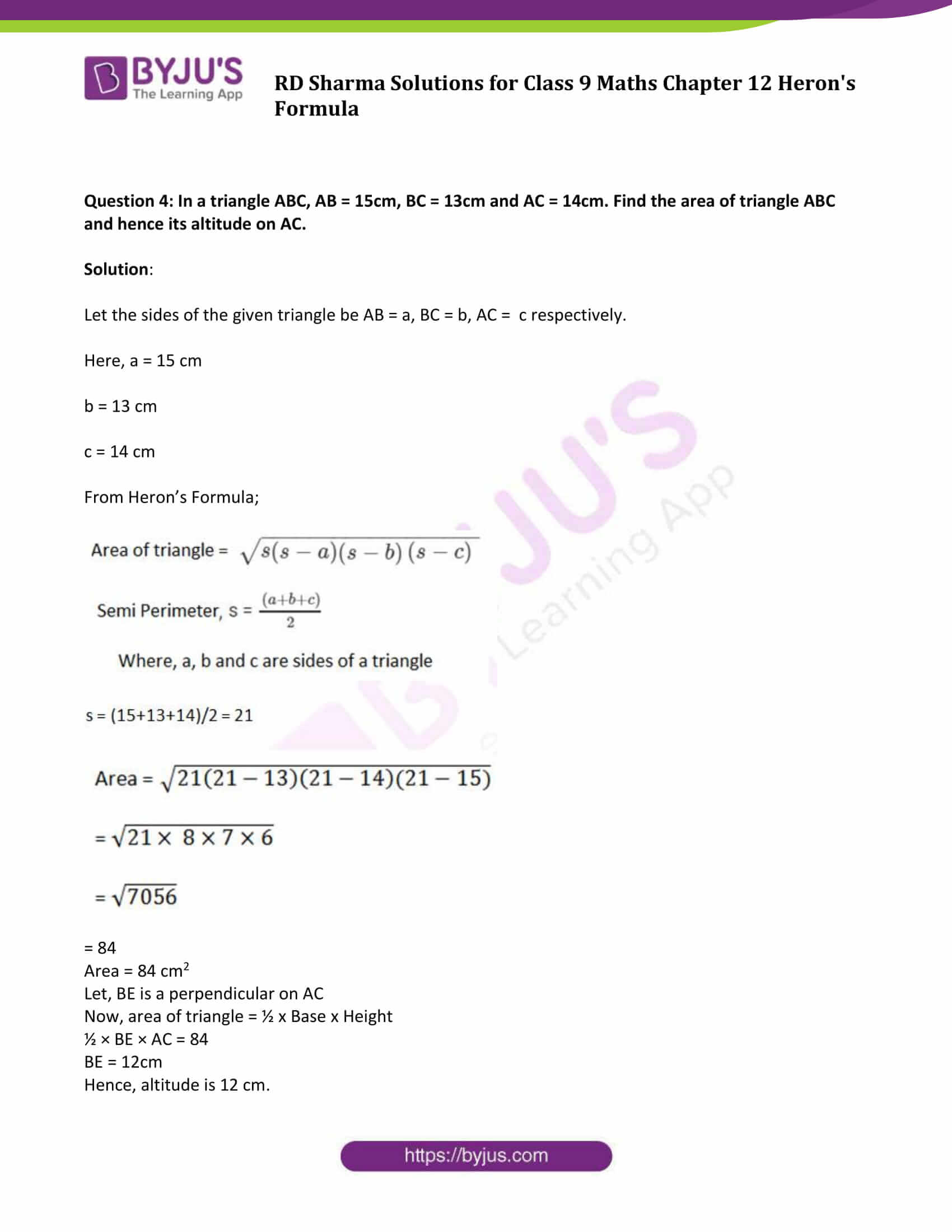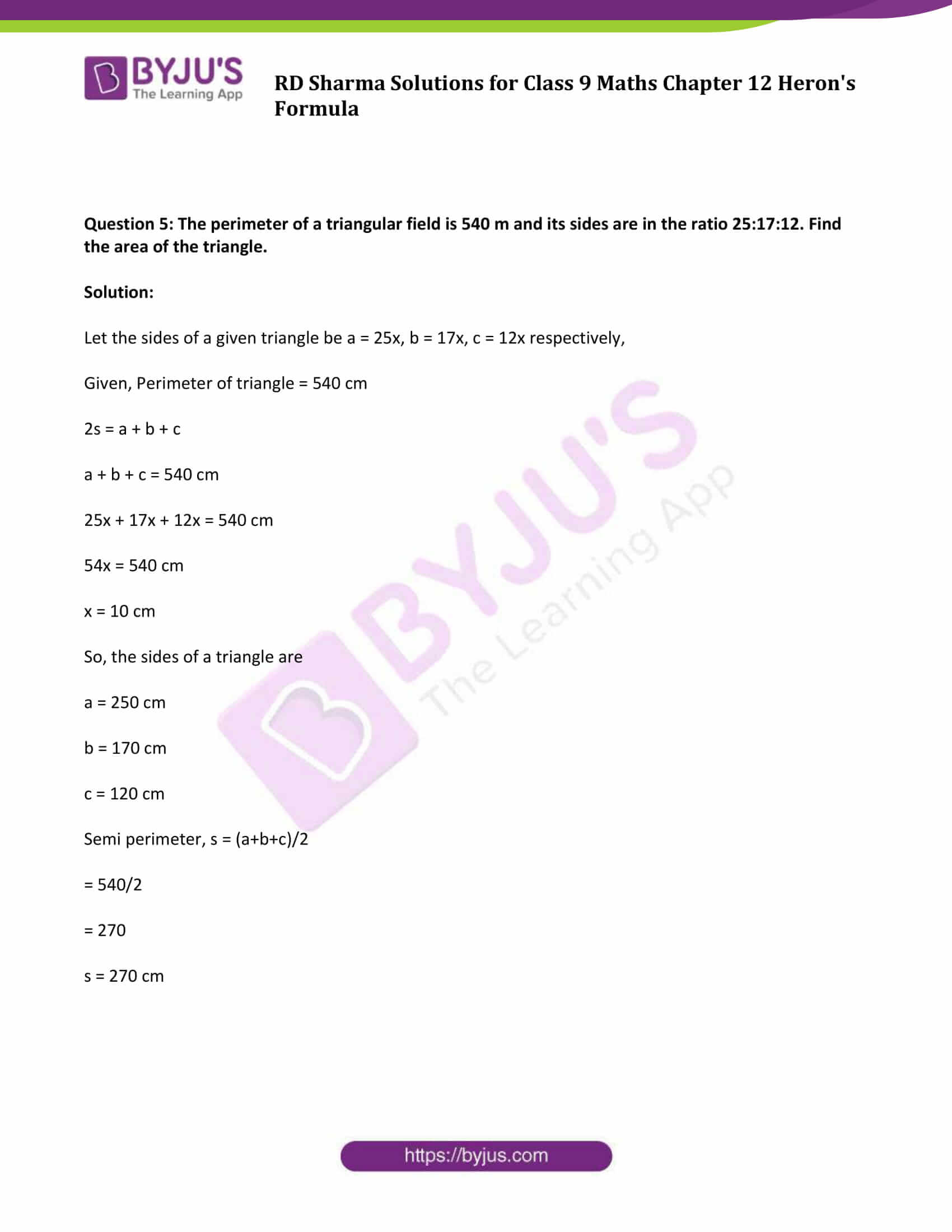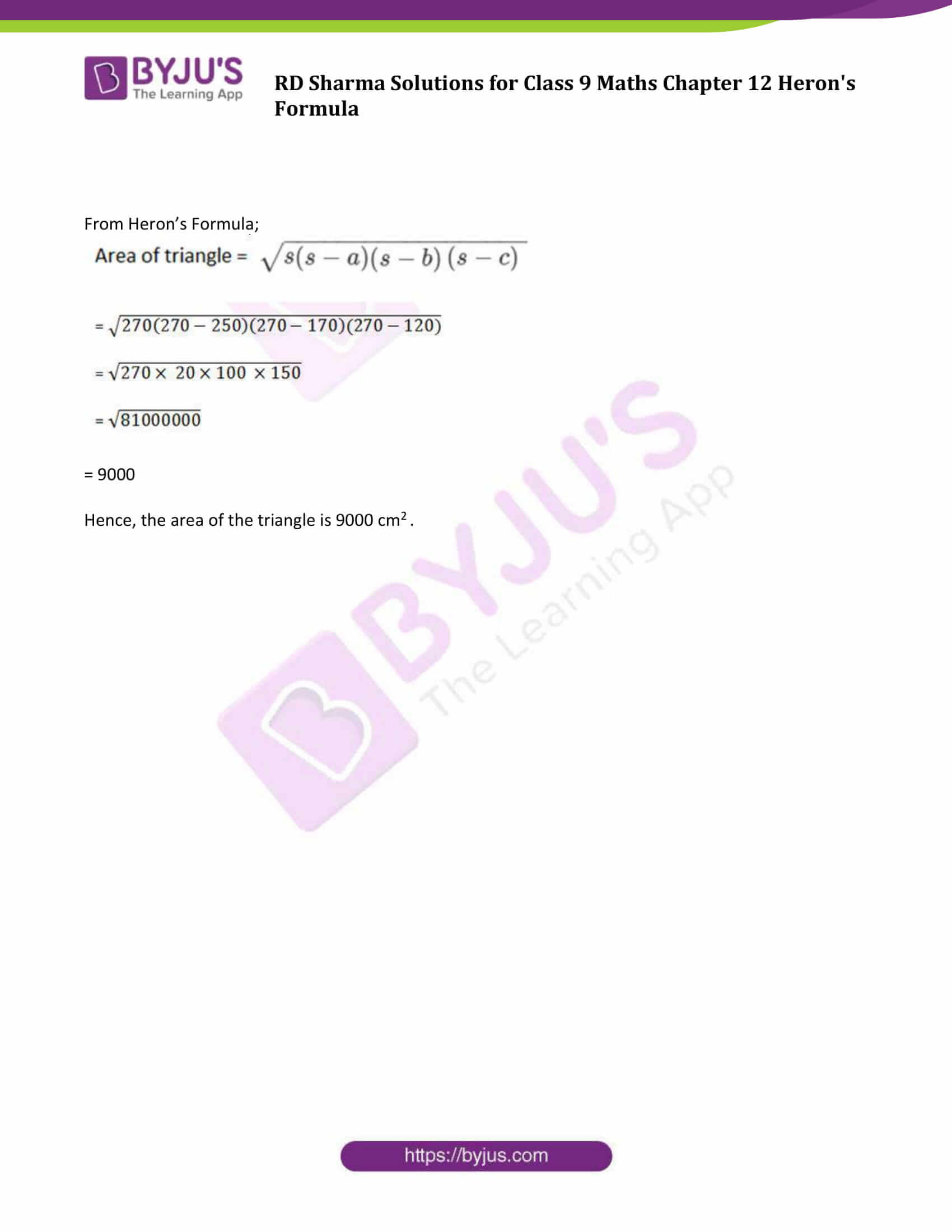### Access Answers to Maths RD Sharma Class 9 Chapter 12 Heron’s Formula Exercise 12.1 Page number 12.8

Question 1: Find the area of a triangle whose sides are respectively 150 cm, 120 cm and 200 cm.

Solution:

We know, Heron’s Formula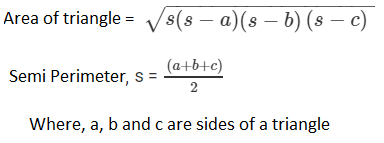Here, a = 150 cm

b = 120 cm

c = 200 cm

Step 1: Find s

s = (a+b+c)/2

s = (150+200+120)/2

s = 235 cm

Step 2: Find Area of a triangle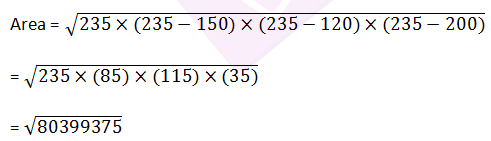= 8966.56

Area of triangle is 8966.56 sq.cm.

Question 2: Find the area of a triangle whose sides are respectively 9 cm, 12 cm and 15 cm.

Solution:

We know, Heron’s FormulaHere, a = 9 cm

b = 12 cm

c = 15 cm

Step 1: Find s

s = (a+b+c)/2

s = (9 + 12 + 15)/2

s = 18 cm

Step 2: Find Area of a triangle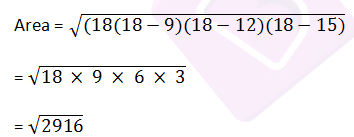= 54

Area of triangle is 54 sq.cm.

Question 3: Find the area of a triangle two sides of which are 18 cm and 10 cm and the perimeter is 42 cm.

Solution:

Given:

a = 18 cm, b = 10 cm, and perimeter = 42 cm

Let c be the third side of the triangle.

Step 1: Find third side of the triangle, that is c

We know, perimeter = 2s,

2s = 42

s = 21

Again, s = (a+b+c)/2

Put the value of s, we get

21 = (18+10+c)/2

42 = 28 + c

c = 14 cm

Step 2: Find area of triangle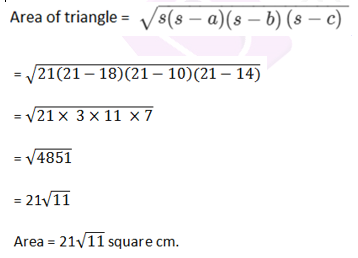Question 4: In a triangle ABC, AB = 15cm, BC = 13cm and AC = 14cm. Find the area of triangle ABC and hence its altitude on AC.

Solution:

Let the sides of the given triangle be AB = a, BC = b, AC = c respectively.

Here, a = 15 cm

b = 13 cm

c = 14 cm

From Heron’s Formula;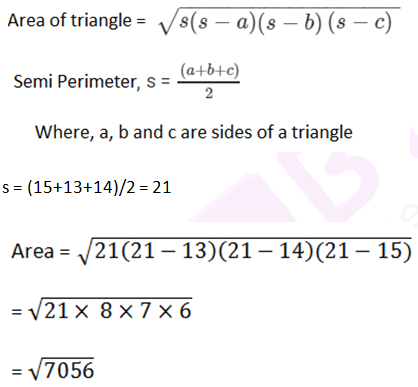= 84

Area = 84 cm2

Let, BE is a perpendicular on AC

Now, area of triangle = ½ x Base x Height

½ × BE × AC = 84

BE = 12cm

Hence, altitude is 12 cm.

Question 5: The perimeter of a triangular field is 540 m and its sides are in the ratio 25:17:12. Find the area of the triangle.

Solution:

Let the sides of a given triangle be a = 25x, b = 17x, c = 12x respectively,

Given, Perimeter of triangle = 540 cm

2s = a + b + c

a + b + c = 540 cm

25x + 17x + 12x = 540 cm

54x = 540 cm

x = 10 cm

So, the sides of a triangle are

a = 250 cm

b = 170 cm

c = 120 cm

Semi perimeter, s = (a+b+c)/2

= 540/2

= 270

s = 270 cm

From Heron’s Formula;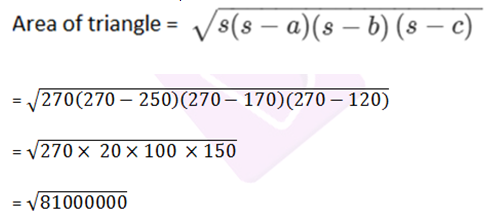= 9000

Hence, the area of the triangle is 9000 cm2 .

## RD Sharma Solutions for Class 9 Maths Chapter 12 Exercise 12.1

Class 9 Maths Chapter 12 Heron’s Formula Exercise 12.1 is based on Heron’s formula. Find detailed RD Sharma solutions for class 9 Maths chapter 12, solved by BYJU’S experts using step-by-step problem solving method here. Students can freely download RD Sharma solutions and sharpen their skills.